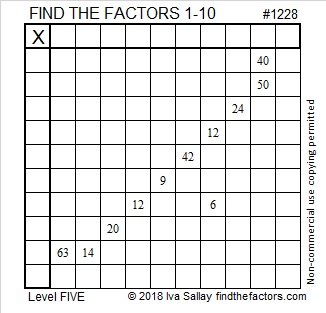# 1228 and Level 5

This level 5 puzzle has a row and a column with the exact same two clues. That ISN’T a good place to start this puzzle! Nevertheless, you can solve it, if you use logic and your knowledge of a basic 10 × 10 multiplication table. There is only one solution. Good luck!Print the puzzles or type the solution in this excel file: 10-factors-1221-1231

Now I’ll share some information about the number 1228:

• 1228 is a composite number.
• Prime factorization: 1228 = 2 × 2 × 307, which can be written 1228 = 2² × 307
• The exponents in the prime factorization are 2 and 1. Adding one to each and multiplying we get (2 + 1)(1 + 1) = 3 × 2  = 6. Therefore 1228 has exactly 6 factors.
• Factors of 1228: 1, 2, 4, 307, 614, 1228
• Factor pairs: 1228 = 1 × 1228, 2 × 614, or 4 × 307
• Taking the factor pair with the largest square number factor, we get √1228 = (√4)(√307) = 2√307 ≈ 35.042831228 is repdigit 444 in BASE 17 because 4(17² + 17 + 1) = 4(307) = 1228